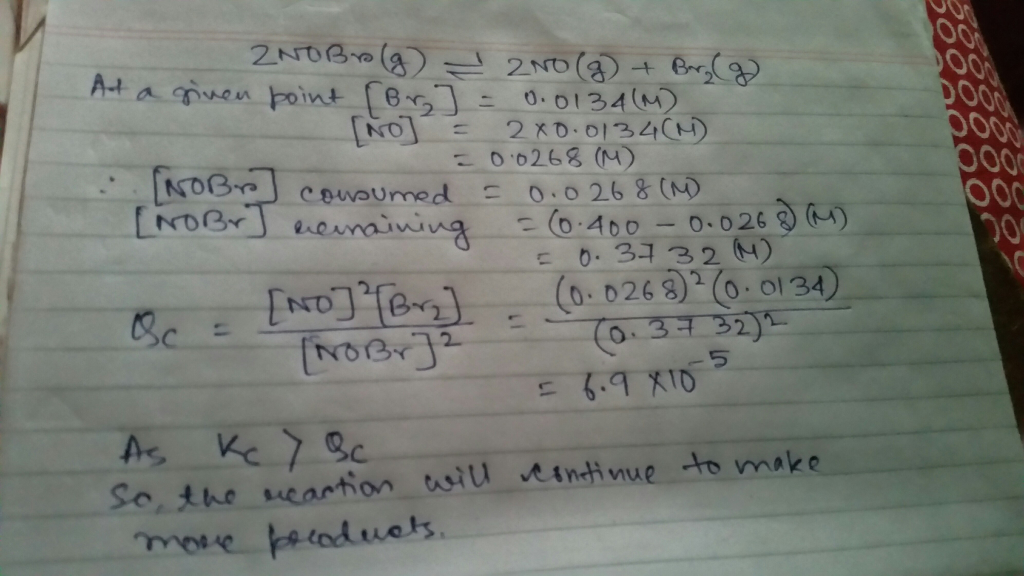# Question & Answer: For the equilibrium 2 NOBr(g) D 2 NO(g) + Br2(g), Kc = 1.56 ´ 10-3 at 300 K. Suppose 0.400…..

For the equilibrium 2 NOBr(g) D 2 NO(g) + Br2(g), Kc = 1.56 ´ 10-3 at 300 K. Suppose 0.400 M NOBr is placed in a reaction vessel at 300 K. At a given point, the concentration of Br2 is 0.0134 M. Which of the following statements is true? The value of K will decrease until it is equal to Q. Q < K so the reaction will continue to make more products. Q > K so the reaction will consume products to make more reactants. The value of K will increase until it is equal to Q. Q = K so the system is at equilibrium.